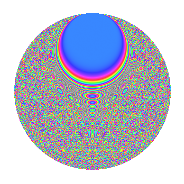# Properties

 Label 2015.2.euLevel 2015 Weight 2 Character orbit eu Rep. character $$\chi_{2015}(324,\cdot)$$ Character field $$\Q(\zeta_{30})$$ Dimension 1760 Sturm bound 448

# Related objects

## Defining parameters

 Level: $$N$$ = $$2015 = 5 \cdot 13 \cdot 31$$ Weight: $$k$$ = $$2$$ Character orbit: $$[\chi]$$ = 2015.eu (of order $$30$$ and degree $$8$$) Character conductor: $$\operatorname{cond}(\chi)$$ = $$2015$$ Character field: $$\Q(\zeta_{30})$$ Sturm bound: $$448$$

## Dimensions

The following table gives the dimensions of various subspaces of $$M_{2}(2015, [\chi])$$.

Total New Old
Modular forms 1824 1824 0
Cusp forms 1760 1760 0
Eisenstein series 64 64 0

## Trace form

 $$1760q - 456q^{4} - 248q^{9} + O(q^{10})$$ $$1760q - 456q^{4} - 248q^{9} + 6q^{10} - 12q^{14} - 392q^{16} - 12q^{25} + 14q^{26} - 80q^{29} - 132q^{30} + 10q^{35} + 832q^{36} + 32q^{39} + 44q^{40} + 152q^{49} - 108q^{51} - 60q^{55} + 220q^{56} - 240q^{61} - 360q^{64} - 42q^{65} - 32q^{66} - 44q^{69} + 44q^{74} + 72q^{75} + 56q^{79} + 156q^{81} + 16q^{90} + 30q^{91} - 48q^{94} - 62q^{95} + O(q^{100})$$

## Decomposition of $$S_{2}^{\mathrm{new}}(2015, [\chi])$$ into irreducible Hecke orbits

The newforms in this space have not yet been added to the LMFDB.